# Calculus 3 : Cylindrical Coordinates

## Example Questions

1 2 18 19 20 21 22 23 24 26 Next →

### Example Question #251 : Cylindrical Coordinates

Express the three-dimensional (x,y,z) Cartesian coordinates as cylindrical coordinates (r, θ, z):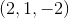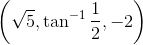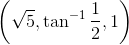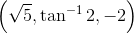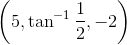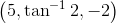Explanation:

The coordinates (2, 1, -2) corresponds to: x = 2, y = 1, z = -2, and are to be converted to the cylindrical coordinates in form of (r, θ, z), where: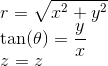So, filling in for x, y, z: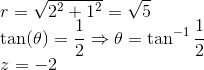Then the cylindrical coordinates are represented as:### Example Question #251 : Cylindrical Coordinates

Express the three-dimensional (x,y,z) Cartesian coordinates as cylindrical coordinates (r, θ, z):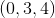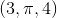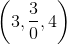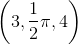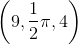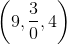Explanation:

The coordinates (0, 3, 4) corresponds to: x = 0, y = 3, z = 4, and are to be converted to the cylindrical coordinates in form of (r, θ, z), where:So, filling in for x, y, z: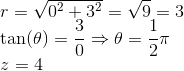Then the cylindrical coordinates are represented as:### Example Question #252 : Cylindrical Coordinates

Express the three-dimensional (x,y,z) Cartesian coordinates as cylindrical coordinates (r, θ, z):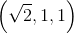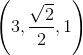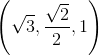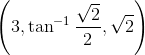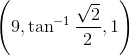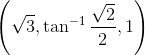Explanation:

The coordinates (√2, 1, 1) corresponds to: x = √2, y = 1, z = 1, and are to be converted to the cylindrical coordinates in form of (r, θ, z), where:So, filling in for x, y, z: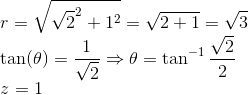Then the cylindrical coordinates are represented as:### Example Question #253 : Cylindrical Coordinates

Express the three-dimensional cylindrical coordinates (r, θ, z) as three-dimensional (x,y,z) Cartesian coordinates: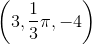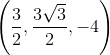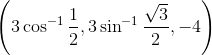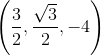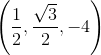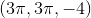Explanation:

The coordinates (3, π/3, -4) corresponds to: r = 3, θ = π/3, z = -4, and are to be converted to the Cartesian coordinates in form of (x, y, z), where: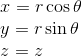So, filling in for r, θ, z: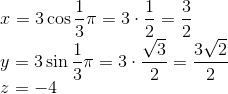Then the Cartesian coordinates are represented as:### Example Question #254 : Cylindrical Coordinates

Express the three-dimensional Cartesian coordinates (x,y,z) as three-dimensional cylindrical coordinates (r, θ, z):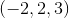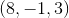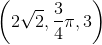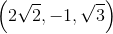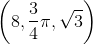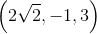Explanation:

The coordinates (-2, 2, 3) corresponds to: x = -2, y = 2, z = 3, and are to be converted to the cylindrical coordinates in form of (r, θ, z), where:So, filling in for x, y, z: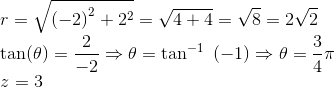Then the cylindrical coordinates are represented as:### Example Question #255 : Cylindrical Coordinates

Express the three-dimensional cylindrical coordinates (r, θ, z) as three-dimensional (x,y,z) Cartesian coordinates: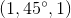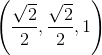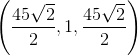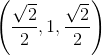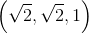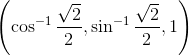Explanation:

The coordinates (1, 45°, 1) corresponds to: r = 1, θ = 45°, z = 1, and are to be converted to the Cartesian coordinates in form of (x, y, z), where:So, filling in for r, θ, z: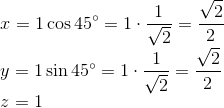Then the Cartesian coordinates are represented as:### Example Question #256 : Cylindrical Coordinates

Express the three-dimensional cylindrical coordinates (r, θ, z) as three-dimensional (x,y,z) Cartesian coordinates: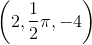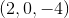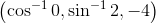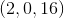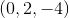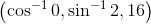Explanation:

The coordinates (2, π/2, -4) corresponds to: r = 2, θ = π/2, z = -4, and are to be converted to the Cartesian coordinates in form of (x, y, z), where:So, filling in for r, θ, z: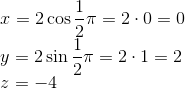Then the Cartesian coordinates are represented as:1 2 18 19 20 21 22 23 24 26 Next →

### All Calculus 3 Resources